Introduction to the polynomial chaos

Welcome back to the uncertainty quantification (UQ) series. In the opening post, we learned what UQ was in the first place, and why you should know it. We also went through the typical workflow involved in the process.

The second post was then about finding and quantifying the significant sources of uncertainty. For example, uncertain material parameters. We saw that ideally, we would know the joint probability distribution of the uncertain variables under consideration. We also saw this is often impossible. In such a case, estimated parameters (mean, variance) can help, as can estimated distributions like histograms. For several random variables and random fields, estimated marginal distributions and covariances can be a life-saver.

However, knowing the characteristics of the input data is not enough. Obviously, it’s the output that ultimately interests us. And to get that, we need a tool model the propagation of uncertainty from the inputs, and to the output.

Enter polynomial chaos.

### Polynomial WHAT??

Yeah, my thougths exactly. The name is really confusing and stupid, and the Wikipedia entry is not exactly helpful either.

But, the idea is quite simple really.

Let’s say we have a random conductivity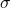. The idea of polynomial chaos is to writeas a weighted sum of polynomials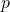of a variable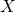: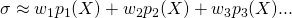The catch is here: the variableis a random variable with a nice distribution. It can be normal, it can be uniform, or it can be any other of the textbook distributions.

The polynomialsare then selected so that all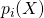are non-correlated. Or, in more mathematical terms, they are orthogonal with respect to‘s distribution. For instance, Hermite polynomials are used with normal distributions, and Legendre polynomials with uniform ones.

The polynomials have some nice properties. The first one is always a constant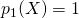. All the others have a zero expected value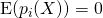.

And like I already mentioned, they all all mutually uncorrelated, meaning that the covariance goes to zero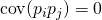,

whenever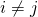. Finally, all of them have have a unit variance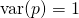,

except for the constant one of course.

#### Simple example

An example might clarify things. Let’s say our conductivity is normally distributed with a mean value of 5, and a variance of 2. (A very realistic example, I know.)

Then, it can be expressed simply as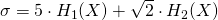,

where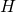denote the aforementioned Hermite polynomials.

The first one takes care of the mean value of. Makes sense right,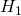being the only polynomial with a non-zero mean. Then, the variance is determined purely by the second term.

Still don’t believe me? Checking the Wikipedia article reveals that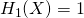and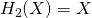, so the above expression is simplified into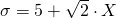.

This indeed has the correct distribution.

#### Interpretation

Now, note one important thing. There’s a direct correspondence between the conductivity, and the normally-distributed random variable. Fix, and the value ofis fixed too.

Indeed, we could as well write thatis a function of: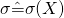.

This is hugely beneficial, as we shall soon see. But first, let’s deal with one issue first.

#### Non-standard distributions

Yeah. What ifdoes not follow any standard distributions?

For example, it might look kinda normal, but with the tails cut off. I mean, you simply can’t have negative conductivities in reality, but a normal distribution would allow this.

In this case, it might be a good idea to use anthat follows the standard uniform distribution. Correspondingly, the polynomials would be of the Legendre type. But finally, each value ofwould have to related to a particular value of.

We can do this with basic high-school statistics principles, by transforming distributions. For example, the lower limit forof course has to correspond to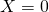. This is fairly straightforward – neither can get any lower. Likewise,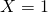would be mapped to the highest possible.

This approach can be followed further. Medians are mapped to medians, and quartiles to quartiles. Just like in the magnificent piece of art below.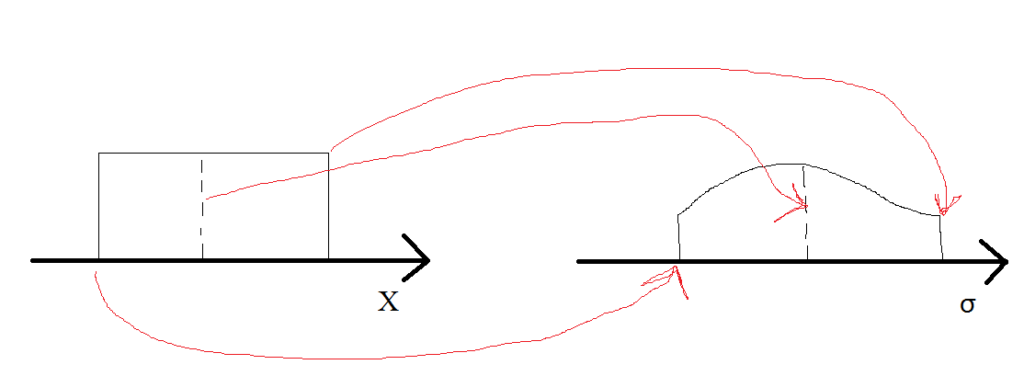You probably see now where this is going. We get an exact 1-on-1 correspondence by using the cumulative distribution functions. Simply put, for any possible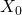, we get the corresponding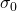by requiring that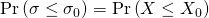.

Once we have this relationship, we can again write the series approximation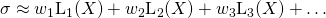with the Legendre polynomials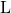. The coefficients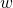can then be determined with linear regression, or the Galerkin’s method. But more of that later.

### Why polynomial chaos?

There’s one really nice benefit to the polynomial chaos approach. Representing the input side uncertainty is kinda meh. We could do that in a million other ways, too.

The real benefit comes from analysing the output. The torque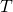of an electrical machine, for instance.

Once we have the input – the conductivity– represented as a series, we do the exact same thing for the output too. We write the torque as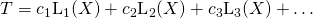,

and determine the coefficients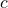with one of the ways described later in this series.

And that’s where the real benefit appears. First of all, the above expression is really easy to analyse. We can determine mean values, variances, distributions, and you-say-what very easily indeed. No need for time-consuming computer simulations any more – just simpl-ish arithmetics.

There’s also another benefit, maybe even more important than the first one. Realize we’re writing both the input and output as function(als) of,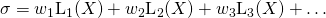This means we have an almost direct expression from the input, to the output. This, in turn, can offer some very useful insights to the effecthas on. Like how any kind of variation in the former influence the latter.

#### Why the same polynomials

Aaaand the mathematical onslaught continues a little bit more. Saw how we’re using the same Legendre polynomialsto represent both the inputand the output?

That’s partly because of maths – the orthogonality of the polynomials is nice.

But, there’s also a more intuitive reason to this. For the input side, we chose to usebecause the conductivitywas roughly uniformly distributed. Hence, choosingto be uniformly distributed as well allowed us to easily get a good polynomial approximation for.

This is exactly why we’re using the same polynomials for representing the output.

Think of it.

If the input is strictly limited to a certain interval, it is almost certain that the output is as well. Hence, an uniformly distributedmakes sense for it too.

Likewise, if the input could obtain any possible value, but were still centered near its mean, the output would probably exhibit the same behaviour. Most of the time, the results would be quite the same, but occasionally you’d get an outlier. In this case, using a normally distributedwould make sense.

### Conclusion

Polynomial chaos enables us to express random variables in a nice concise form. That alone may not be very important in itself. Its real power comes from establishing a relationship between the random input, and the output. That way, the quantity of interest can be analysed very easily, without time-consuming simulations.

So far, we’ve only considered a single random variable. Next time, we shall see how several random variables, or random functions can also be handled with the polynomial chaos approach.

Until then!

-Antti

Need help with electric motor design or design software? Let's get in touch - satisfaction guaranteed!Uncertainty and Maths – What is Polynomial Chaos